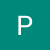# Foundations of Data Science

Nowadays, all of us have heard that “Data is the new oil and the Data Science is the combustion engine that drives it” or “Data Science is the most sought after job of the twenty-first century” or “Data Science is the future”. In this article, we discuss what exactly is Data Science.

## What is Data Science?

Before we start answering this question, it’s important to understand why there is so much confusion(something so popular) around it, why there is no clear understanding of what data science is.

One reason for this is that it’s an assortment of several tasks, there are different tasks involved…

# Operations on NumPy arrays

In the previous article, we discussed how to index the NumPy arrays. In this article, we discuss how to perform operations on NumPy arrays. Let’s get started.

Let’s create some sample arrays of the same size to play around with, the good thing with NumPy is that we can treat the arrays as vectors and we can perform operations on top of them just like with vectors.

For example, we can perform the addition of two arrays simply with the ‘+’ operator and it will do the element-wise addition of two arrays.

Let’s do the same thing using random numbers…

# High dimensional array and Creating NumPy array

In the last article, we started with the NumPy package and discussed its advantages, the performance it offers. In this article, we discuss how to create a NumPy array.

Let’s just visualize what the high-dimensional array looks like. In the picture below, we have an array that contains 4 numbers, so this is a 1-D array with 4 numbers

We can use 1-D arrays to represent things like time-series data for instance what is the temperature per hour, it becomes a 1-D array.

If we expand it one dimension, it becomes a 2-D array wherein the below case there are…

# How to describe relationships between variables?

In the last article, we discussed how a histogram could help us understand the data distribution for an attribute. In this article, we discuss how to understand the relationship between multiple attributes.

# Scatter Plots

This is a common observation in many real-world datasets where for a given object, we have several attributes describing the object so for example in the Sports domain, in Cricket, we have the Runs scored, Balls faced, Minutes played, Strike Rate, Type of dismissal. …

# Stem and Leaf plots

In the last article, we looked at the histogram as a way of describing and understanding the trends in Quantitative Data. Let’s look at another kind of plot for describing Quantitative Data which is the Stem and Leaf plot. It is an efficient way of describing small to medium data sets.

Let’s say we have the data for the runs scored by Sachin in his last 30 ODIs.

Stem and Leaf plot represents every number/data item in the data(score in each match in this case) in two parts, one is called the stem and the other is called the leaf

# Uses of Histograms in Machine Learning

In the last few articles, we have discussed histogram in great detail, and it's clear by now that histogram reveals a lot of trends in the data, it helps us understand interesting patterns in the data. Histograms are also used in Machine Learning for various purposes and in this article, we discuss the uses of histograms in Machine Learning. So, let’s get started.

1. Identifying Discriminatory features

Let’s say we are trying to build a machine learning system that takes a lot of information about a patient such as Age, Height, Weight, Cholesterol, Sugar Level, and so on and this Machine…

# Typical trends in Histogram

The main purpose when we draw a histogram is to see if some trends are visible in the data or not and there are some standard trends that we should look out for in the histogram. These standard trends are discussed in this article. So, let’s get started.

Here is the list of standard trends:

1. To look for how far are the values in the data spread out
2. Is the data density high in certain intervals — if data is divided into class intervals, is it the case that there are few intervals that have very tall bars and the…

# Histogram

In the previous article, we looked at how Histogram is the preferred way of representing Quantitative Data and how to select the perfect bin size. In this article, we look at how to answer questions on percentages /proportions— “In what percentage of matches did Sachin score less than 10 runs?”. As with the case with a frequency table, frequency plot, questions related to percentages are a bit hard to answer directly from the histogram.

Below is a histogram of runs scored by Sachin Tendulkar in all the ODIs he played taking a bin size of 10

Suppose we want to…

# How to Describe Quantitative Data?

Before we get started with this question, the primary question that we are guided with when describing Qualitative Data is “What is the frequency of different categories?” and we plotted the frequencies using Frequency Table, Frequency Plots, Relative Frequencies Plots, and so on.